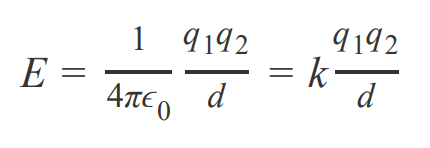# Problem: Calculate the amount of energy released in the formation of one mole of BaSe bonds (not lattice energy). The radius of the barium ion is 1.35 Å, and the radius of the selenide ion is 1.98 Å. Note that 1 Å = 10−10 m.Atoms involved in bonds are usually more stable than they would be otherwise. This means that energy is released in the formation of the bond. For ionic bonds, Coulomb's law is used to calculate this energy:where E is energy in joules (J), k is a constant equal to 1/(4πϵ0) = 8.99 × 109 (J ⋅ m)/C2, q1 and q2 are the charges of the two ions, and dd is the distance between the nuclei of the two ions in meters (m). The charges on the ions (q1 and q2) are expressed in units of electrical charge, coulombs (C), and are calculated using the charge on the ion and the charge on an electron (1.60 × 10− 19 C).While the bond energy calculated by Coulomb's law pertains to a single ionic bond, lattice energy pertains to all the ionic bonding among a group of atoms arranged in a crystal lattice. Lattice energy is calculated by using the Coulomb's law equation, but with a different constant (unique to each substance) that takes into account the crystalline structure of the substance.By convention, lattice energy is defined as the amount required to either break an ionic solid into individual gaseous ions, or to form an ionic solid from gaseous ions.

###### FREE Expert Solution
81% (8 ratings)View Complete Written Solution
###### Problem Details

Calculate the amount of energy released in the formation of one mole of BaSe bonds (not lattice energy). The radius of the barium ion is 1.35 Å, and the radius of the selenide ion is 1.98 Å. Note that 1 Å = 10−10 m.

Atoms involved in bonds are usually more stable than they would be otherwise. This means that energy is released in the formation of the bond. For ionic bonds, Coulomb's law is used to calculate this energy:where E is energy in joules (J), k is a constant equal to 1/(4πϵ0) = 8.99 × 109 (J ⋅ m)/C2, q1 and q2 are the charges of the two ions, and dd is the distance between the nuclei of the two ions in meters (m). The charges on the ions (q1 and q2) are expressed in units of electrical charge, coulombs (C), and are calculated using the charge on the ion and the charge on an electron (1.60 × 10− 19 C).

While the bond energy calculated by Coulomb's law pertains to a single ionic bond, lattice energy pertains to all the ionic bonding among a group of atoms arranged in a crystal lattice. Lattice energy is calculated by using the Coulomb's law equation, but with a different constant (unique to each substance) that takes into account the crystalline structure of the substance.

By convention, lattice energy is defined as the amount required to either break an ionic solid into individual gaseous ions, or to form an ionic solid from gaseous ions.